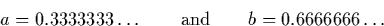Next: Two Suitable Digit Representations Up: Approaches to Exact Real Previous: Approaches to Exact Real

## Representation Problems

When we perform exact real number computations, we may require a representation of infinite size. However when performing computations with structures such as infinite sequences of digits, one problem which is encountered is that there is no least significant' digit. This is because as the representation extends infinitely far to the right. This fact means that arithmetic operations on such sequences must be computed from left (most significant digit) to right (least significant digit). Most operations on floating point numbers, by contrast, require computation from right to left. Floating point addition, for example, is performed by starting with the least significant digits of the input numbers and working towards the most significant.

Given that we must implement arithmetic operations from left to right on streams, it quickly becomes apparent that representations such as binary and decimal are not adequate. Surprisingly, using these representations even simple operations such as addition and subtraction are not computable. For example, suppose we wish to compute the result (a + b) of adding two numbers which start as follows:It is not clear whether the first digit of the result here should be a one or a zero. If, for example, the numbers continued as shown here until 100th digit of a, but at this point the next digit of a was greater than three then the result of the calculation would be greater than one. If the same digit in fact turned out to be less than three, the result would be less than one. In general it is not possible to compute an output digit without examining an infinite number of digits. Similar examples show that all integer bases suffer from the same problem.

In order to find algorithms for exact real number computation using infinite objects, we must use different representations. The representations used in this work are described next, and we also mention a number of other possible representations. Note that all the representations used for exact real arithmetic are equivalent to one another in the sense that it is possible to convert between them in a computable way. Some representations may be more efficient than others.

It is only possible to represent an countable subset of the real numbers in a computer using streams--the so called `computable reals''.Next: Two Suitable Digit Representations Up: Approaches to Exact Real Previous: Approaches to Exact Real
Martin Escardo
5/11/2000